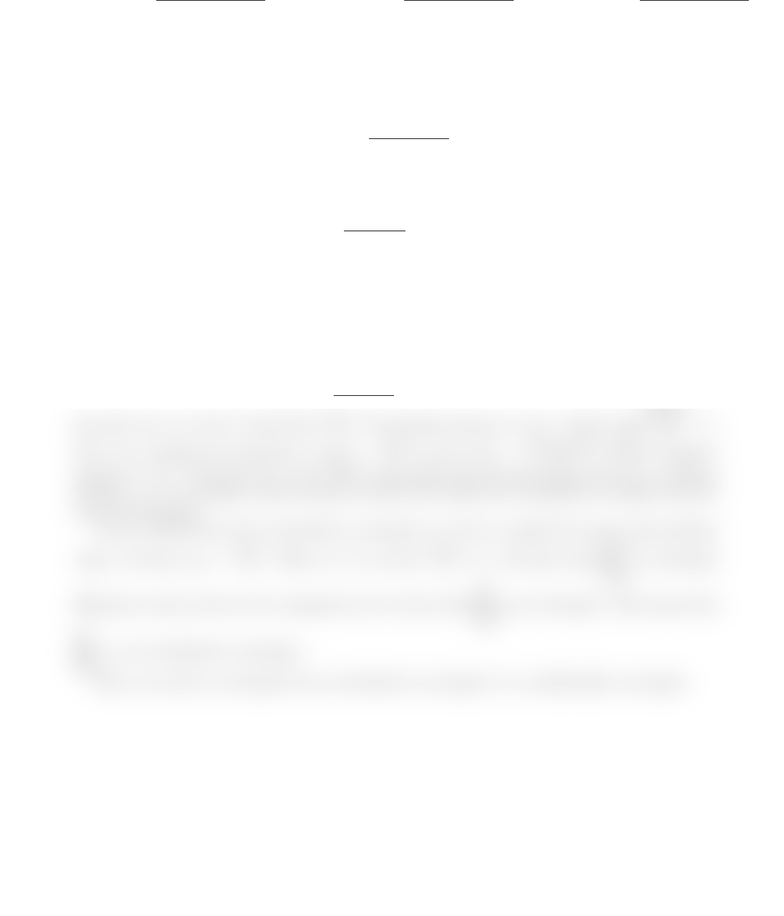Study Guides (380,000)
CA (150,000)
U of A (4,000)
MATH (300)
Quiz

# MATH214 Study Guide - Quiz Guide: Alternating Series Test, Ratio Test, Alternating Series

Department
Mathematics
Course Code
MATH214
Professor
Pierre Youssef
Study Guide
Quiz

This preview shows half of the first page. to view the full 2 pages of the document.Quiz #2.
First Name: Family Name: ID Number:
Do not speak to or communicate with other students. Calculators, textbooks, notes are not allowed.
Problem 1. Is the following series convergent? Is it absolutely convergent? Is it conditionally
convergent?
X
n=3
(1)nln2(n)
n
Problem 2. Find the radius and interval of convergence of the following series:
X
n=3
22nln2(n)
(3)n·n(x3/4)n
Solutions
Problem 1. The general term is an=(1)nln2(n)
n. This is an alternating series. To use the alternat-
ing series test, we need to check that ln2(n)
nis decreasing and goes to zero. Clearly lim
n→∞
ln2(n)
n= 0.
Now if we calculate the derivative of f(x) = ln2(x)
xwe get f0(x) = ln(x)·(2ln(x))
x2which is negative
whenever x>e2. Therefore for n>9, ln2(n)
nis decreasing. By the alternating series test, we deduce
that Pn>9anis convergent. Since the ﬁrst 8 terms won’t aﬀect the convergence we deduce that the
series is convergent.
Now to check if the series is absolutely convergent, we need to consider the series of the absolute
values. We have |an|=ln2(n)
n. When n>3, we have ln2(n)
n>1
n. We know that
X
n=3
1
nis divergent
(Harmonic series), then by the comparison test we have that
X
n=3
|an|is divergent. This means that
X
n=3
anis not absolutely convergent.
Since our series is convergent but not absolutely convergent, it is conditionally convergent.